# RD Sharma Solutions for Class 8 Maths Chapter 6 - Algebraic Expressions and Identities Exercise 6.7

RD Sharma Solutions for Class 8 Maths Exercise 6.7 of Chapter 6 Algebraic Expressions and Identities are provided in simple pdf format. From the exam point of view, BYJU’S experts have solved and prepared these solutions which help students gain mathematical skills to excel in their board exams. Regularly, practising the RD Sharma Solutions helps students score high marks in their exams. The pdf’s can be downloaded easily from the links given below. In Exercise 6.7 of Chapter 6 algebraic expressions and identities, we shall study problems based on a special product.

## Download the pdf of RD Sharma Solutions For Class 8 Maths Exercise 6.7 Chapter 6 Algebraic Expressions and Identities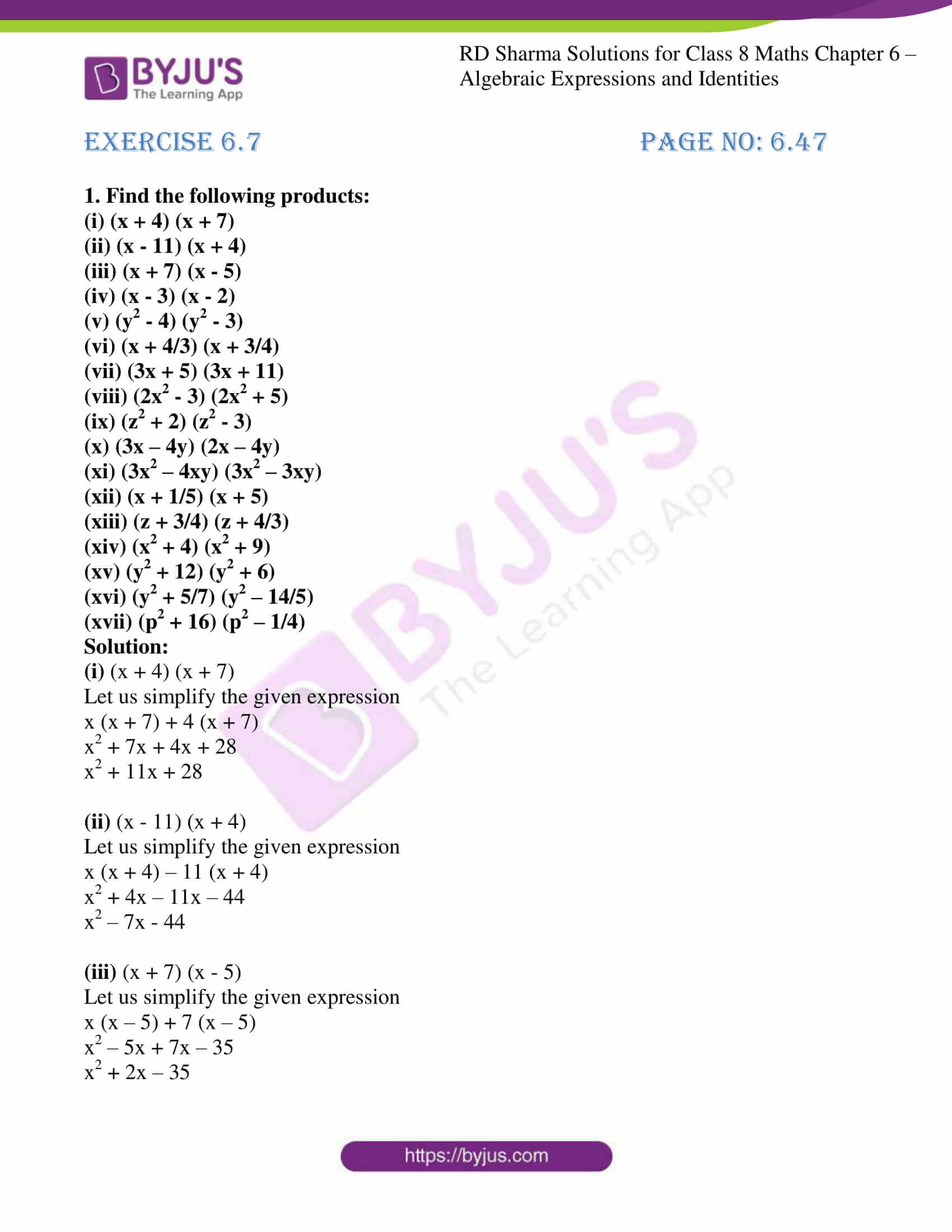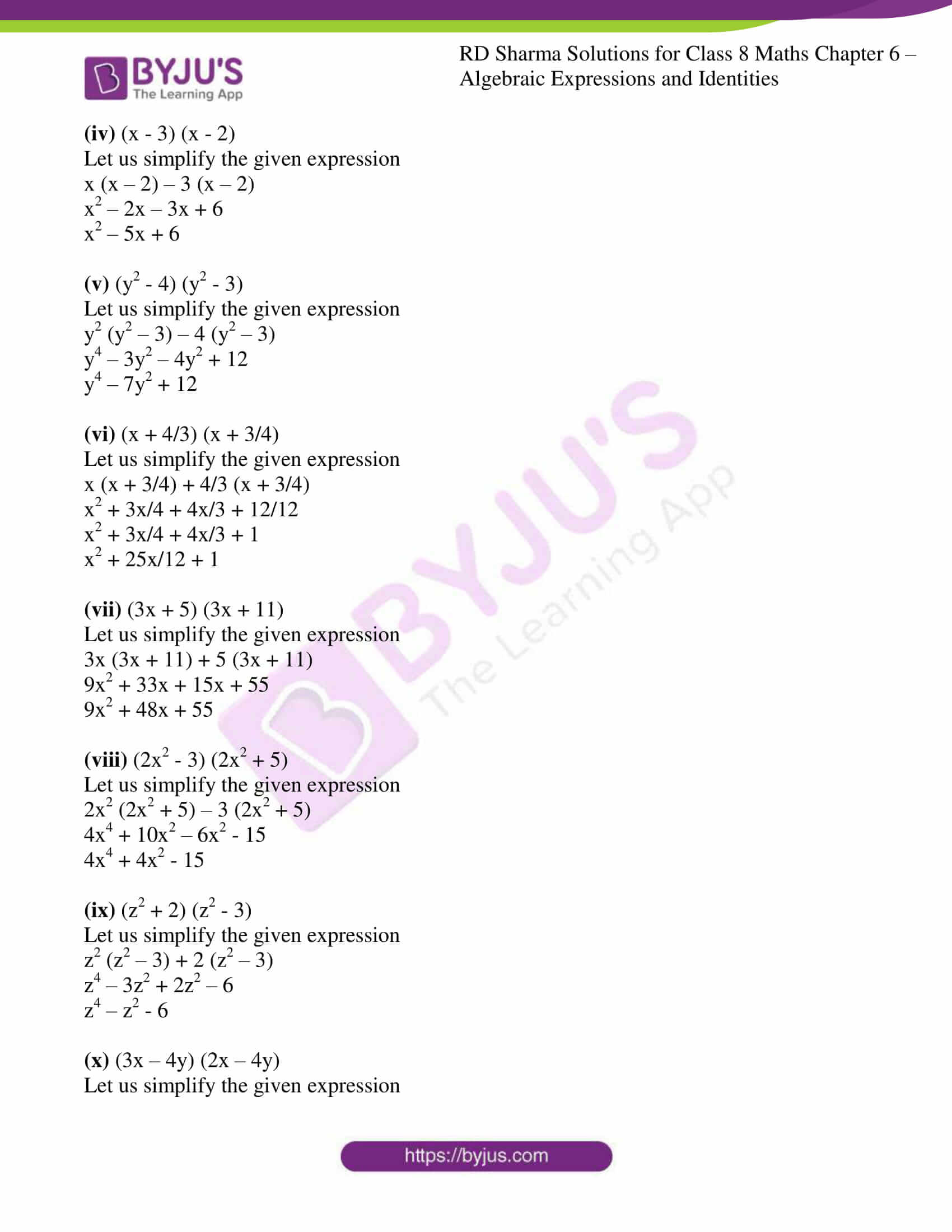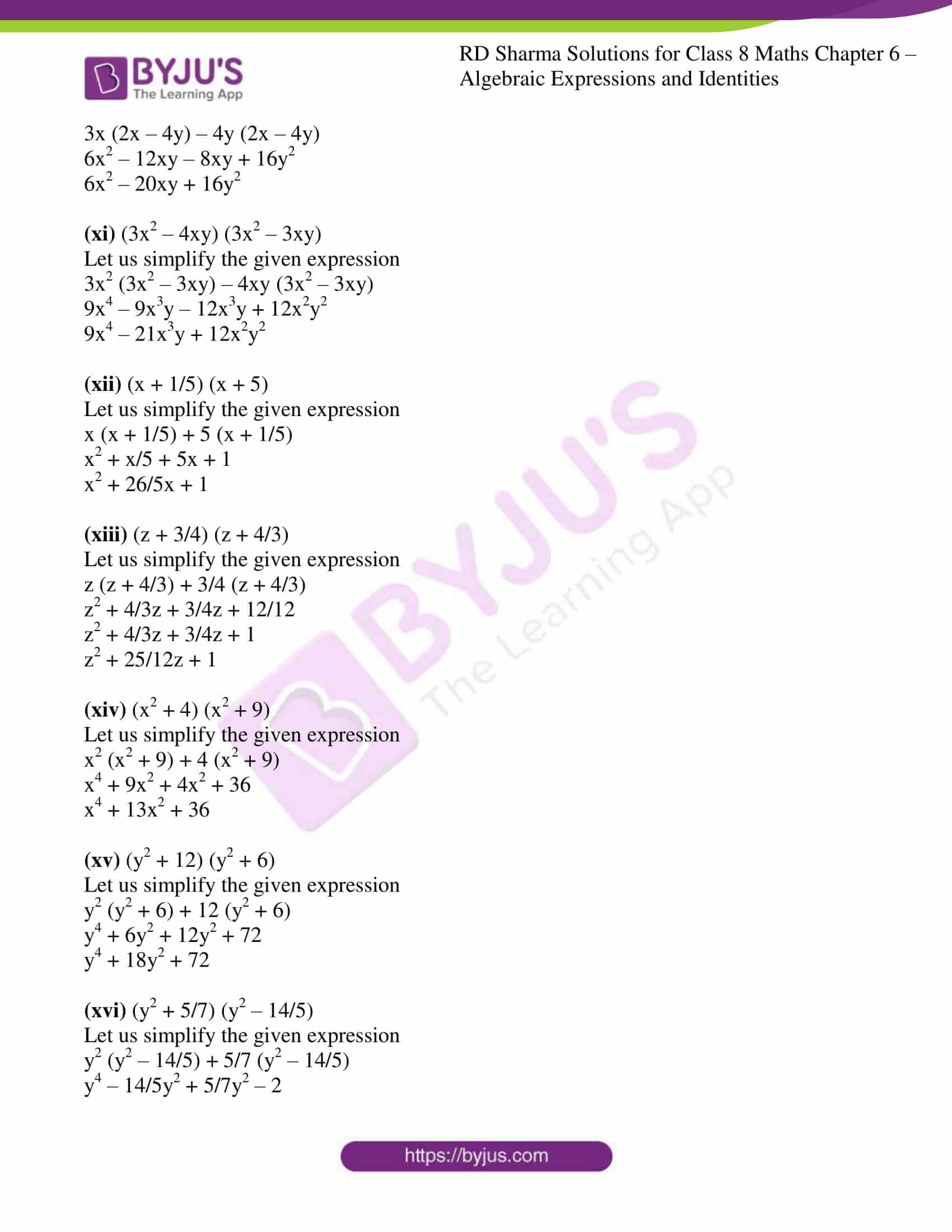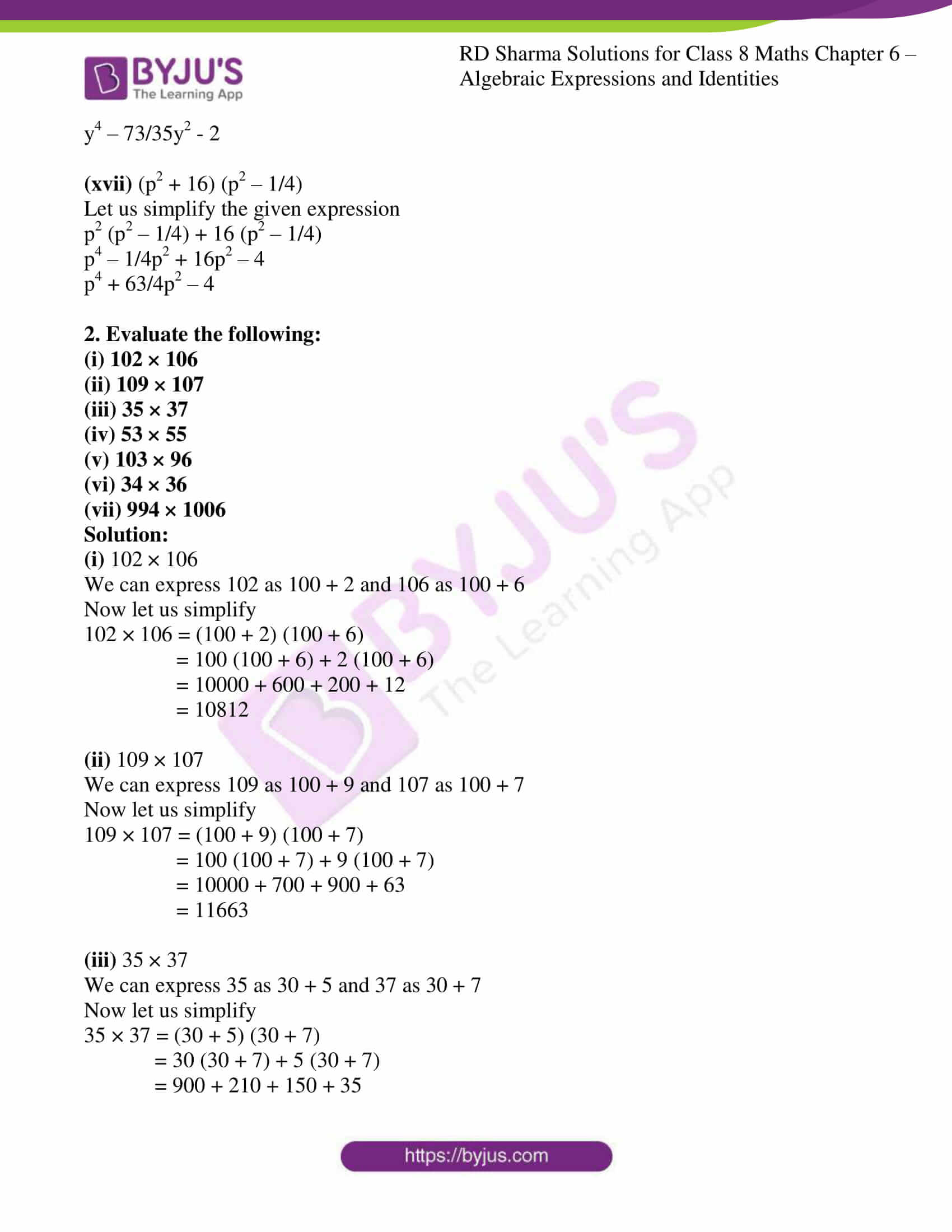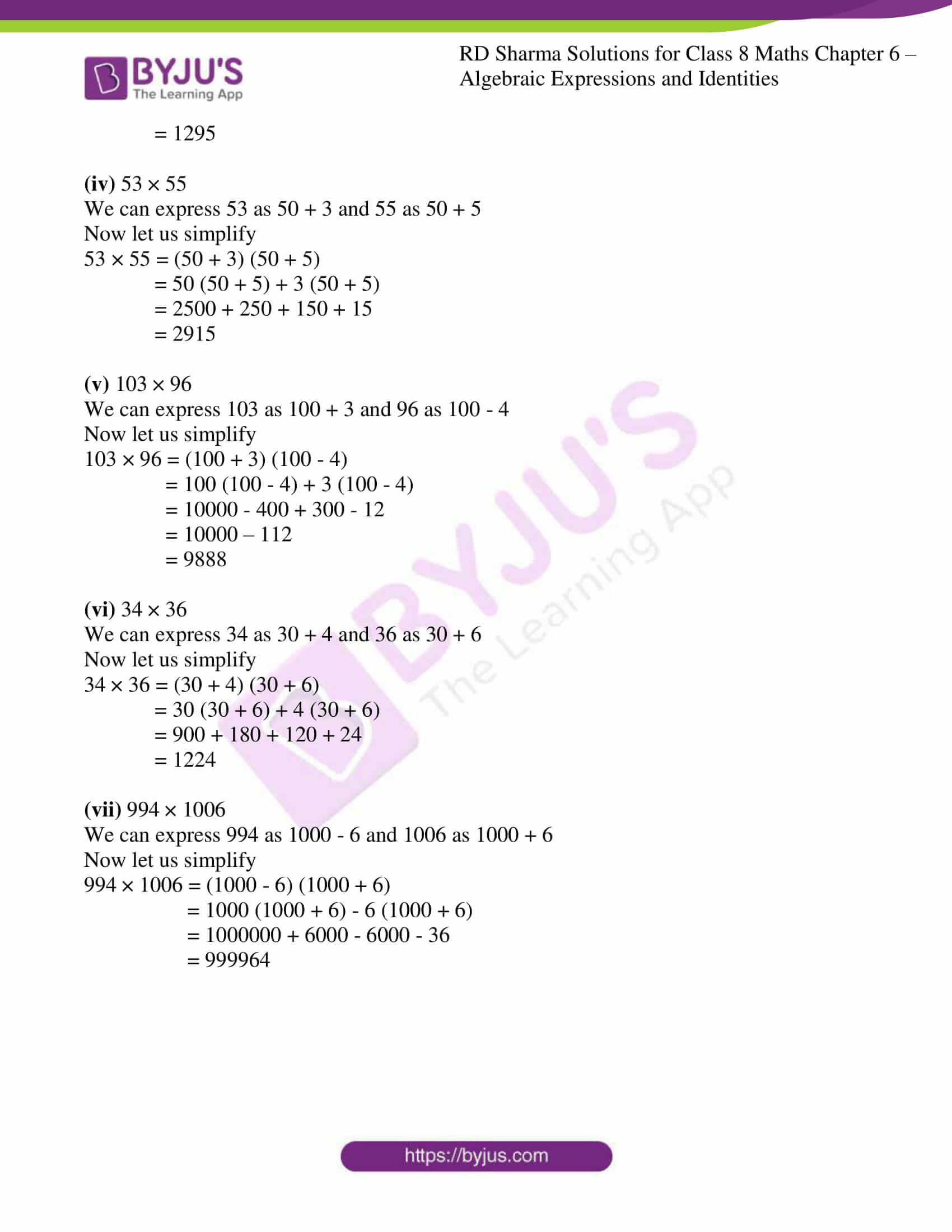### Access Answers to RD Sharma Solutions for Class 8 Maths Exercise 6.7 Chapter 6 Algebraic Expressions and Identities

#### EXERCISE 6.7 PAGE NO: 6.47

1. Find the following products:

(i) (x + 4) (x + 7)

(ii) (x – 11) (x + 4)

(iii) (x + 7) (x – 5)

(iv) (x – 3) (x – 2)

(v) (y2 – 4) (y2 – 3)

(vi) (x + 4/3) (x + 3/4)

(vii) (3x + 5) (3x + 11)

(viii) (2x2 – 3) (2x2 + 5)

(ix) (z2 + 2) (z2 – 3)

(x) (3x – 4y) (2x – 4y)

(xi) (3x2 – 4xy) (3x2 – 3xy)

(xii) (x + 1/5) (x + 5)

(xiii) (z + 3/4) (z + 4/3)

(xiv) (x2 + 4) (x2 + 9)

(xv) (y2 + 12) (y2 + 6)

(xvi) (y2 + 5/7) (y2 – 14/5)

(xvii) (p2 + 16) (p2 – 1/4)

Solution:

(i) (x + 4) (x + 7)

Let us simplify the given expression

x (x + 7) + 4 (x + 7)

x2 + 7x + 4x + 28

x2 + 11x + 28

(ii) (x – 11) (x + 4)

Let us simplify the given expression

x (x + 4) – 11 (x + 4)

x2 + 4x – 11x – 44

x2 – 7x – 44

(iii) (x + 7) (x – 5)

Let us simplify the given expression

x (x – 5) + 7 (x – 5)

x2 – 5x + 7x – 35

x2 + 2x – 35

(iv) (x – 3) (x – 2)

Let us simplify the given expression

x (x – 2) – 3 (x – 2)

x2 – 2x – 3x + 6

x2 – 5x + 6

(v) (y2 – 4) (y2 – 3)

Let us simplify the given expression

y2 (y2 – 3) – 4 (y2 – 3)

y4 – 3y2 – 4y2 + 12

y4 – 7y2 + 12

(vi) (x + 4/3) (x + 3/4)

Let us simplify the given expression

x (x + 3/4) + 4/3 (x + 3/4)

x2 + 3x/4 + 4x/3 + 12/12

x2 + 3x/4 + 4x/3 + 1

x2 + 25x/12 + 1

(vii) (3x + 5) (3x + 11)

Let us simplify the given expression

3x (3x + 11) + 5 (3x + 11)

9x2 + 33x + 15x + 55

9x2 + 48x + 55

(viii) (2x2 – 3) (2x2 + 5)

Let us simplify the given expression

2x2 (2x2 + 5) – 3 (2x2 + 5)

4x4 + 10x2 – 6x2 – 15

4x4 + 4x2 – 15

(ix) (z2 + 2) (z2 – 3)

Let us simplify the given expression

z2 (z2 – 3) + 2 (z2 – 3)

z4 – 3z2 + 2z2 – 6

z4 – z2 – 6

(x) (3x – 4y) (2x – 4y)

Let us simplify the given expression

3x (2x – 4y) – 4y (2x – 4y)

6x2 – 12xy – 8xy + 16y2

6x2 – 20xy + 16y2

(xi) (3x2 – 4xy) (3x2 – 3xy)

Let us simplify the given expression

3x2 (3x2 – 3xy) – 4xy (3x2 – 3xy)

9x4 – 9x3y – 12x3y + 12x2y2

9x4 – 21x3y + 12x2y2

(xii) (x + 1/5) (x + 5)

Let us simplify the given expression

x (x + 1/5) + 5 (x + 1/5)

x2 + x/5 + 5x + 1

x2 + 26/5x + 1

(xiii) (z + 3/4) (z + 4/3)

Let us simplify the given expression

z (z + 4/3) + 3/4 (z + 4/3)

z2 + 4/3z + 3/4z + 12/12

z2 + 4/3z + 3/4z + 1

z2 + 25/12z + 1

(xiv) (x2 + 4) (x2 + 9)

Let us simplify the given expression

x2 (x2 + 9) + 4 (x2 + 9)

x4 + 9x2 + 4x2 + 36

x4 + 13x2 + 36

(xv) (y2 + 12) (y2 + 6)

Let us simplify the given expression

y2 (y2 + 6) + 12 (y2 + 6)

y4 + 6y2 + 12y2 + 72

y4 + 18y2 + 72

(xvi) (y2 + 5/7) (y2 – 14/5)

Let us simplify the given expression

y2 (y2 – 14/5) + 5/7 (y2 – 14/5)

y4 – 14/5y2 + 5/7y2 – 2

y4 – 73/35y2 – 2

(xvii) (p2 + 16) (p2 – 1/4)

Let us simplify the given expression

p2 (p2 – 1/4) + 16 (p2 – 1/4)

p4 – 1/4p2 + 16p2 – 4

p4 + 63/4p2 – 4

2. Evaluate the following:

(i) 102 × 106

(ii) 109 × 107

(iii) 35 × 37

(iv) 53 × 55

(v) 103 × 96

(vi) 34 × 36

(vii) 994 × 1006

Solution:

(i) 102 × 106

We can express 102 as 100 + 2 and 106 as 100 + 6

Now let us simplify

102 × 106 = (100 + 2) (100 + 6)

= 100 (100 + 6) + 2 (100 + 6)

= 10000 + 600 + 200 + 12

= 10812

(ii) 109 × 107

We can express 109 as 100 + 9 and 107 as 100 + 7

Now let us simplify

109 × 107 = (100 + 9) (100 + 7)

= 100 (100 + 7) + 9 (100 + 7)

= 10000 + 700 + 900 + 63

= 11663

(iii) 35 × 37

We can express 35 as 30 + 5 and 37 as 30 + 7

Now let us simplify

35 × 37 = (30 + 5) (30 + 7)

= 30 (30 + 7) + 5 (30 + 7)

= 900 + 210 + 150 + 35

= 1295

(iv) 53 × 55

We can express 53 as 50 + 3 and 55 as 50 + 5

Now let us simplify

53 × 55 = (50 + 3) (50 + 5)

= 50 (50 + 5) + 3 (50 + 5)

= 2500 + 250 + 150 + 15

= 2915

(v) 103 × 96

We can express 103 as 100 + 3 and 96 as 100 – 4

Now let us simplify

103 × 96 = (100 + 3) (100 – 4)

= 100 (100 – 4) + 3 (100 – 4)

= 10000 – 400 + 300 – 12

= 10000 – 112

= 9888

(vi) 34 × 36

We can express 34 as 30 + 4 and 36 as 30 + 6

Now let us simplify

34 × 36 = (30 + 4) (30 + 6)

= 30 (30 + 6) + 4 (30 + 6)

= 900 + 180 + 120 + 24

= 1224

(vii) 994 × 1006

We can express 994 as 1000 – 6 and 1006 as 1000 + 6

Now let us simplify

994 × 1006 = (1000 – 6) (1000 + 6)

= 1000 (1000 + 6) – 6 (1000 + 6)

= 1000000 + 6000 – 6000 – 36

= 999964